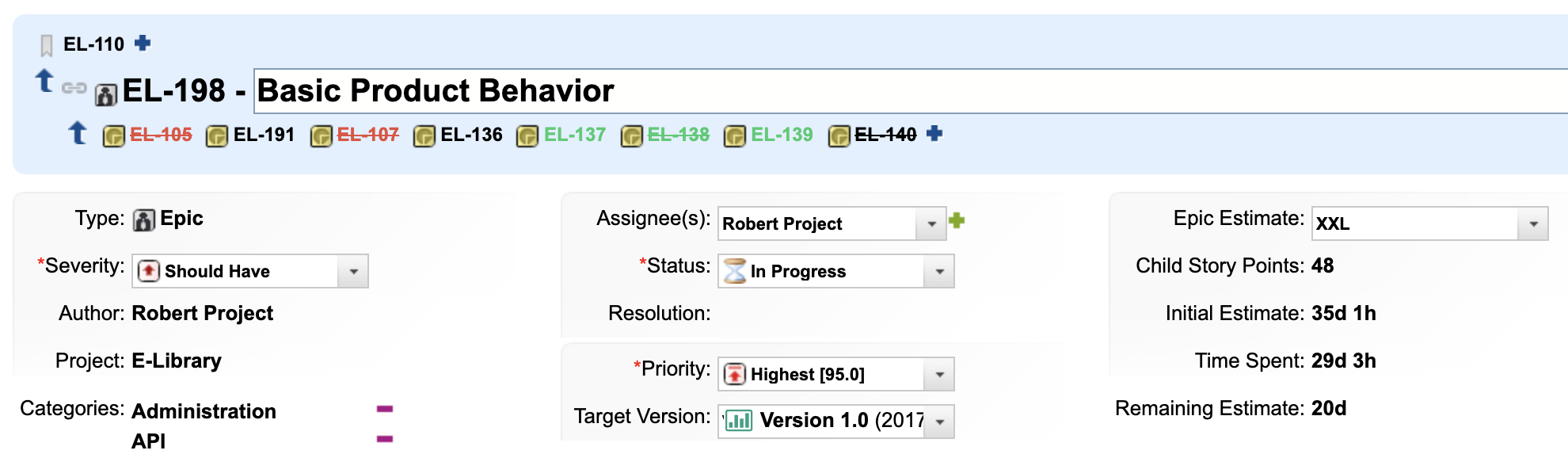# Calculate Epic progress

Modified on Wed, 20 Jan 2021 at 07:31 PM

The goal is to calculate the progress of the epic based on child stories.Let's suppose you have an Epic with User Stories as children like in Elibrary demo project.  So the Initial Estimate, Time Spent & Remaining Estimate fields are derived from (calculated as the sum of) children.Now lets configure the  Nextedy GANTT so it calculates the progress of the epic based on this data. So we want the progress  to be

progress = timeSpent / ( remaniningEstimate + timeSpent)

and

if the item is resolved then  progress = 1

if the item is not resolved and (remainingEstimate + timeSpent)=0 then progress = 0

You can configure gantt according the requirements above using the Widget Properties > Advanced > Item Script

```if(wi.type.id==='epic'){
var all =  ( wi.getRemainingEstimate()!=null ? wi.getRemainingEstimate().getHours() : 0 )  +  ( wi.getTimeSpent()!=null ? wi.getTimeSpent().getHours() : 0 )  ;
var done = ( wi.getTimeSpent()!=null ? wi.getTimeSpent().getHours() : 0 )  ;
if(wi.getResolution()!=null){
}else if(all==0){
}else{
}
var progressString = "Time Spent:" + ( wi.getTimeSpent()!=null ? wi.getTimeSpent() : "0h" )  + " Remaining Estimate: " + ( wi.getRemainingEstimate()!=null ? wi.getRemainingEstimate() : "0h"  )  + " completion: " +  Math.round(task.progress*100) + "%"    ;
}```

If you want to show the info next to the epic, you can also change the label rendered to the right of the epic. You can do it by using Widget Properties > Advanced > Gantt Config Script

```    gantt.templates.rightside_text = function(start, end, task){
};
gantt.config.drag_progress = false;```As a result, the Gantt will now compute the progress of the epic automatically ...